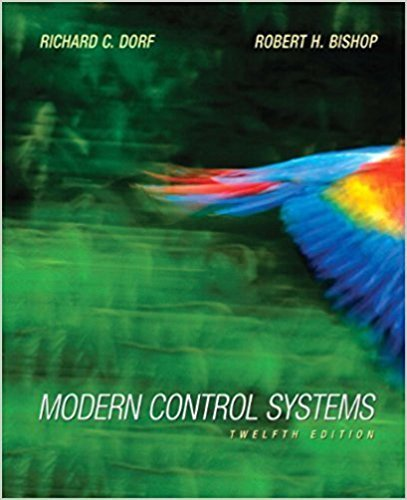Modern Control Systems - 12 Edition - Chapter 4 - Problem P4.4
Register Now

Join StudySoup

Get Full Access to Modern Control Systems - 12 Edition - Chapter 4 - Problem P4.49780136024583

# A control system has two forward paths, as shown in Figure P4.4. (a) Determine the

Modern Control Systems | 12th Edition

Problem P4.4

A control system has two forward paths, as shown in Figure P4.4. (a) Determine the overall transfer function 7(5) = Y(s)/R(s). (b) Calculate the sensitivity, S, using Equation (4.16). (c) Does the sensitivity depend on U(s) or M(*)?

Accepted Solution
Step-by-Step Solution:
Step 1 of 3

Energy Equation ● Ein - Eout + Egen = Estored – Does this refer to a Control Volume Jeff Luttrell MAE 3314 Spring 2016 1 Fourier's Law of Conduction ● In conduction, the flow of heat energy is proportional to the space derivative of the temperature – Heat Flow vs Heat Flux dT qx=−k A Eq. 2.1 dx qx dT q''x= =−k Eq. 2.2 A dx Jeff Luttrell MAE 3314 Spring 2016 2 Newton's Law of Cooling ●In convection, the flow of heat energy is proportional to a temperature difference – Heat Flow vs. Heat Flux q q''=

###### Chapter 4, Problem P4.4 is Solved

Step 2 of 3

Step 3 of 3

Unlock Textbook Solution